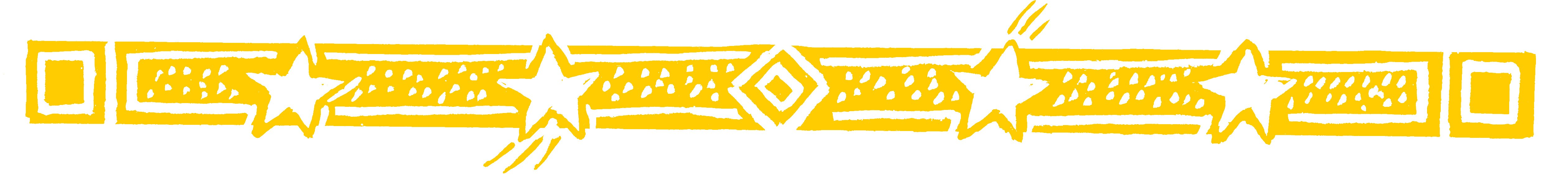ON THE MOTORWAYOn a car journey, get the children to find the digit sum of car number plates.

Any number of any size can always be reduced to a single figure by adding its digits.

• For example 42 has two digits which add up to 6.
We say "the digit sum of 42 is 6".

• The digit sum of 413 is 8 because 4+1+3=8.

• For 20511 the digit sum is 9.

Question Comment !
 Tutorial Title Question Title No Questions 0 Questions Asked 0 Skipped Questions 0 Correctly Answered 0 Wrongly Answered 0 Total Question Attempts 0 Current Question Attempts 0

Skip Question

Reset Test

Halt Test

 No Questions 0 Questions Asked 0 Skipped Questions 0 Correctly Answered 0 Wrongly Answered 0 Total Question Attempts 0

Time Taken

CloseOn The Motorway (1)

• Now suppose we want the digit sum of 417.
Adding 4, 1 and 7 gives 12.
But as 12 is a 2-figure number we add its digits to
get 3 (1+2=3).

We could write  417 = 12 = 3.

• And so 737 = 17 = 8.

We simply add the digits in the number and add again if necessary.

This is simple and one of its uses is in checking sums, as we will see.On The Motorway (2)All things that can be
known have number;
for it is not possible
that without numbers
anything can be either
conceived or known
PHILOLAUS